## 2.3.9 Voter method2.6

The Voter method (also called Hyperdynamics of the infrequent events'') was proposed in Refs. [Voter 97b,Voter 97a] and used to calculate the diffusional processes of Ag atoms on a Ag(111) surface, achieving an acceleration of the calculation up to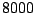times. For the first time we have applied the method to magnetic system and that is the motivation of the work presented in this section.

The method consists in modification of the external potential, basing on the Hessian energy matrix, whereare the system coordinates, so that the transition state (saddle point) remains unchanged. An additional external boost potential,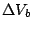(see Fig. 2.21) is slowly switched on at the minimum, rising its value, and is switched off near the transition surface, i.e. where the first eigenvalue of the Hessian matrixbecomes negative. This boost term is always positive making easier to escape from the potential minimum. The Langevin dynamics is then performed in this modified potential. The total time for the escape of the particle from the minimum can be evaluated as the sum of modified times at each timestep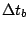, which could be computed from the Langevin dynamics timestep in the modified potential,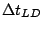, as the following:(2.64)

We have implemented the method for collection of non-interacting magnetic particles with external field applied at some angle to their anisotropy axis. According to the A.Voter's suggestion, we have tried two forms of the boost potentials to accelerate the stochastic dynamical calculations: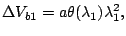(2.65)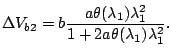(2.66)

Here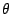is the standard Heaviside function andand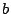are arbitrary parameters which could be tuned. On one hand, these parameters must be as large as possible to achieve the acceleration. On the other hand, the tuning of these parameters could be performed based on the fact that the equilibrium statistics should be achieved in the minimum, i.e. the number of LD time steps performed in the modified potential before the particle is escaped from the minimum must be large enough. The difference between the two potentials is that the second one, although more difficult to evaluate, produces much smoother modification and does not introduce a crest'' in the minimum, from which the particle could be scattered (see Fig. 2.21).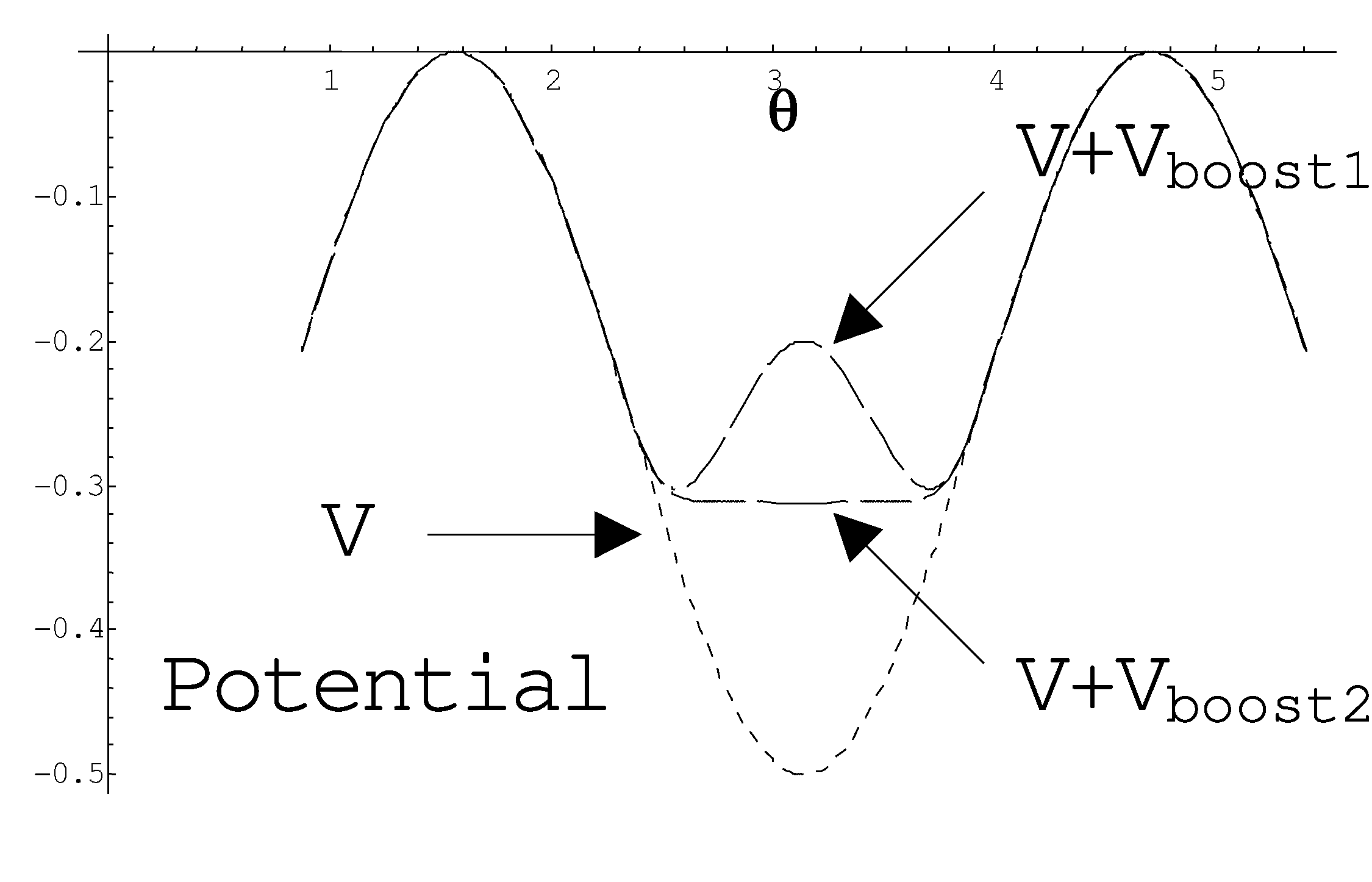The implementation of the method requires the constant evaluation of the lowest eigenvalue of the correspondent Hessian matrix. The direct normal mode analysis is a time consuming procedure which depends strongly on the system size and would limit the acceleration achieved by the method. The direct evaluation of the eigenvalue problem scales like, being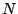the number of moments in the system, whereas the number of operations in a Langevin dynamics steps scales like. In order to obtain a good performance of the method, advanced methods that scale likeare desirable. The iterative methods, such as the Gauss-Siegel, which could use the previous value as initial guess, could be very helpful. A.Voter [Voter 97a] also suggested to replace the direct evaluation ofby its approximate evaluation by means of the numerical minimization (with respect to the parameter s) of the following expression:(2.67)

whereis a small parameter.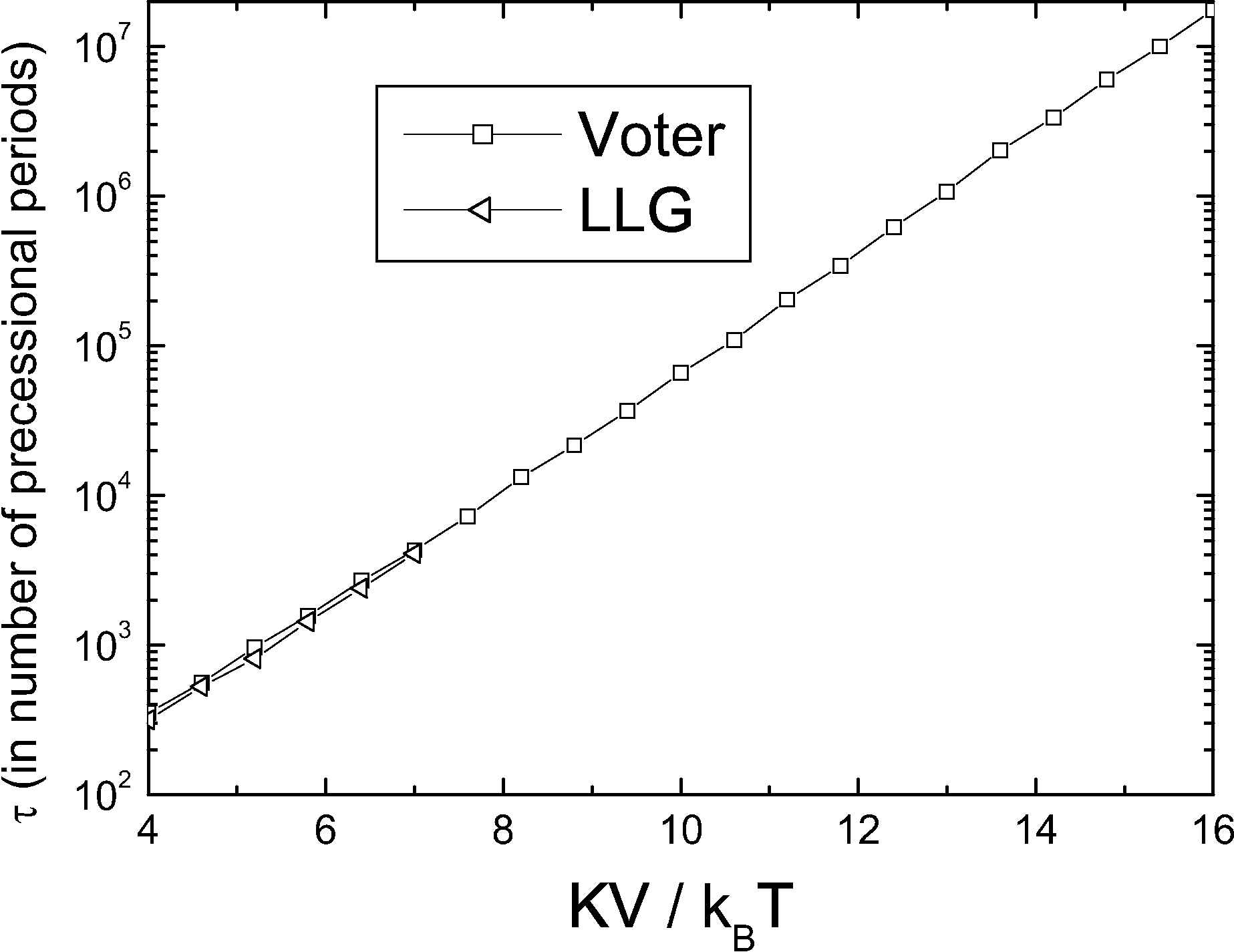The averaged time for the particle to escape from the minimum is calculated using the A.Voter method and compared to that obtained from the direct integration of the LLG equation with a random term representing temperature fluctuations. Fig. 2.22 presents results of the calculations for switching time for an ensemble of uniaxial particles averaged over many realizations. The computation is stopped when the standard deviation from the average value is below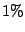. It is clear that Voter's method for reasonable computational time is much faster than the direct LLG integration. Remarkably, the method reproduces correctly all the features of the dynamics, including the precession. Fig. 2.23 presents the histogram for switching time of a particle for energy barrier value. It shows that the accelerated dynamics correctly reproduces the form of adistribution, whereis the reversal time, although the general tendency of the Voter distribution is the displacement to larger values.We have checked the results for different values of the damping parameters and different angles between the applied field and the anisotropy directions. Fig. 2.24 presents the results for an angle between the anisotropy direction and applied field of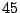degrees and for different values of the tuning parameter. Therefore, unlike the TQMC method (see discussion in Section 2.3.5), the hyperdynamics method correctly reproduces the influence of the ellipticity of the precessional cone on the thermal switching statistics.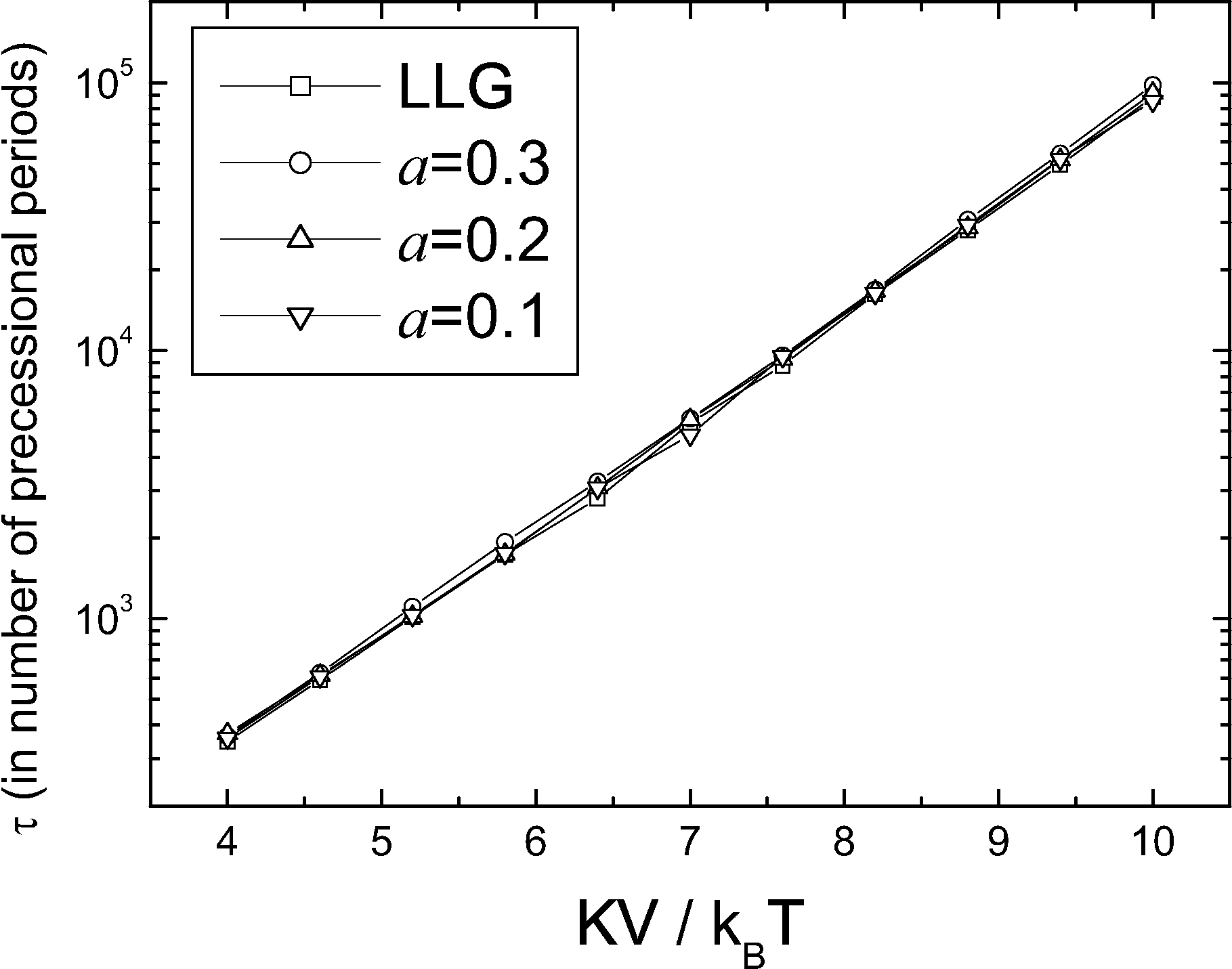The real acceleration of the method depends on the efficiency to calculate the lowest eigenvalue of a complex large system. Therefore, for the small barriers case, the direct integration of the LLG equation is faster. To compare, we present in Fig. 2.25 the ratio between the average CPU time used in the Voter method (using direct lowest eigenvalue evaluation in system described by Eq. (2.67)) and the average CPU time used in the LD dynamics. The acceleration in calculation appears for barrier values larger than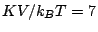. For this straightforward'' implementation, the acceleration up to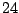times in CPU time has been reached. More sophisticated methods will improve this ratio. The method was also checked for a linear chain of 16 exchange coupled magnetic moments with open boundary condition without dipolar interactions. In this case the Hessian is a banded matrix, being a full matrix with dipolar interactions. The result is shown in Fig. 2.26. The disadvantages of a direct evaluation of the Hessian eigenvalue problem matrix become evident even in this relatively simple system.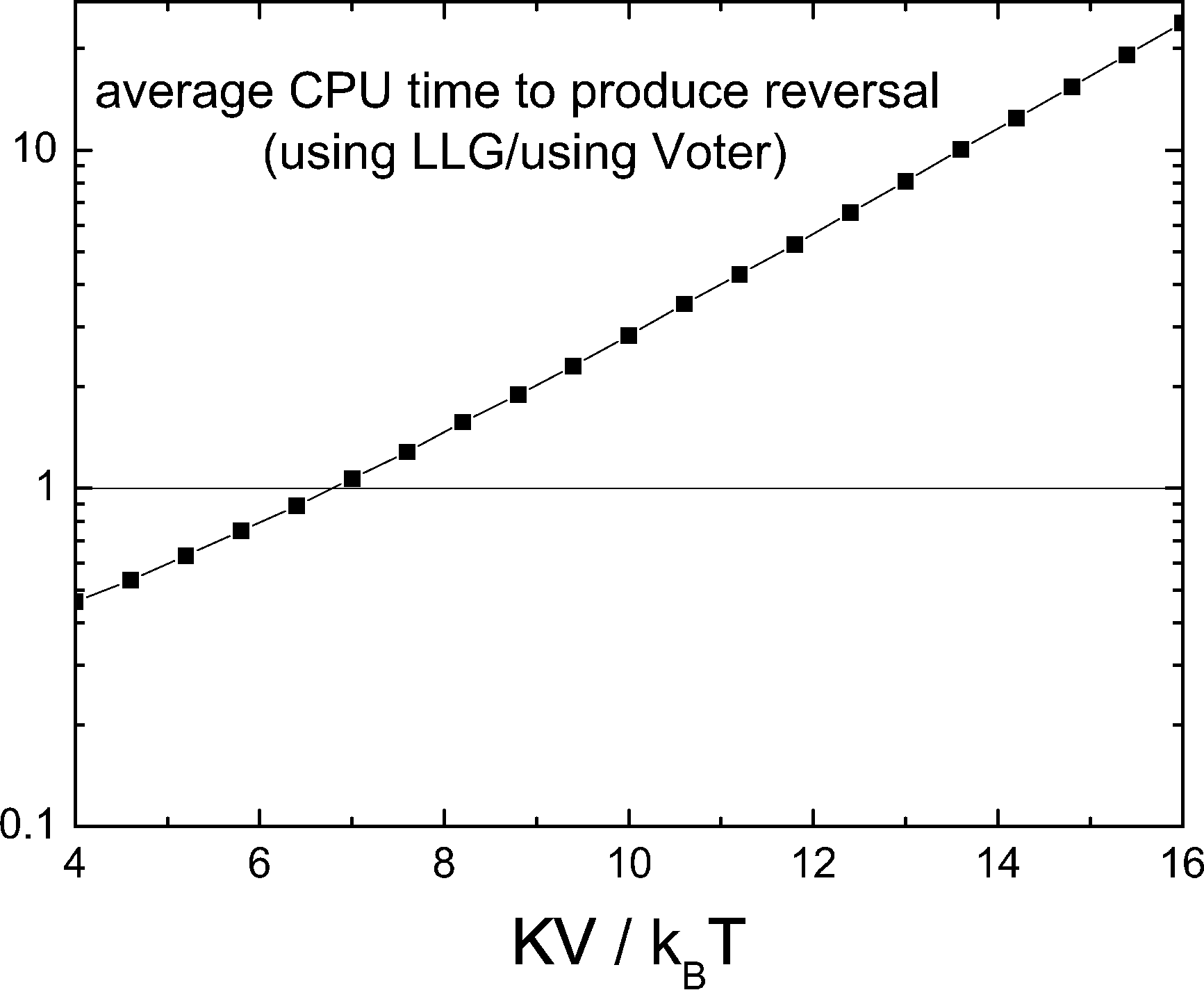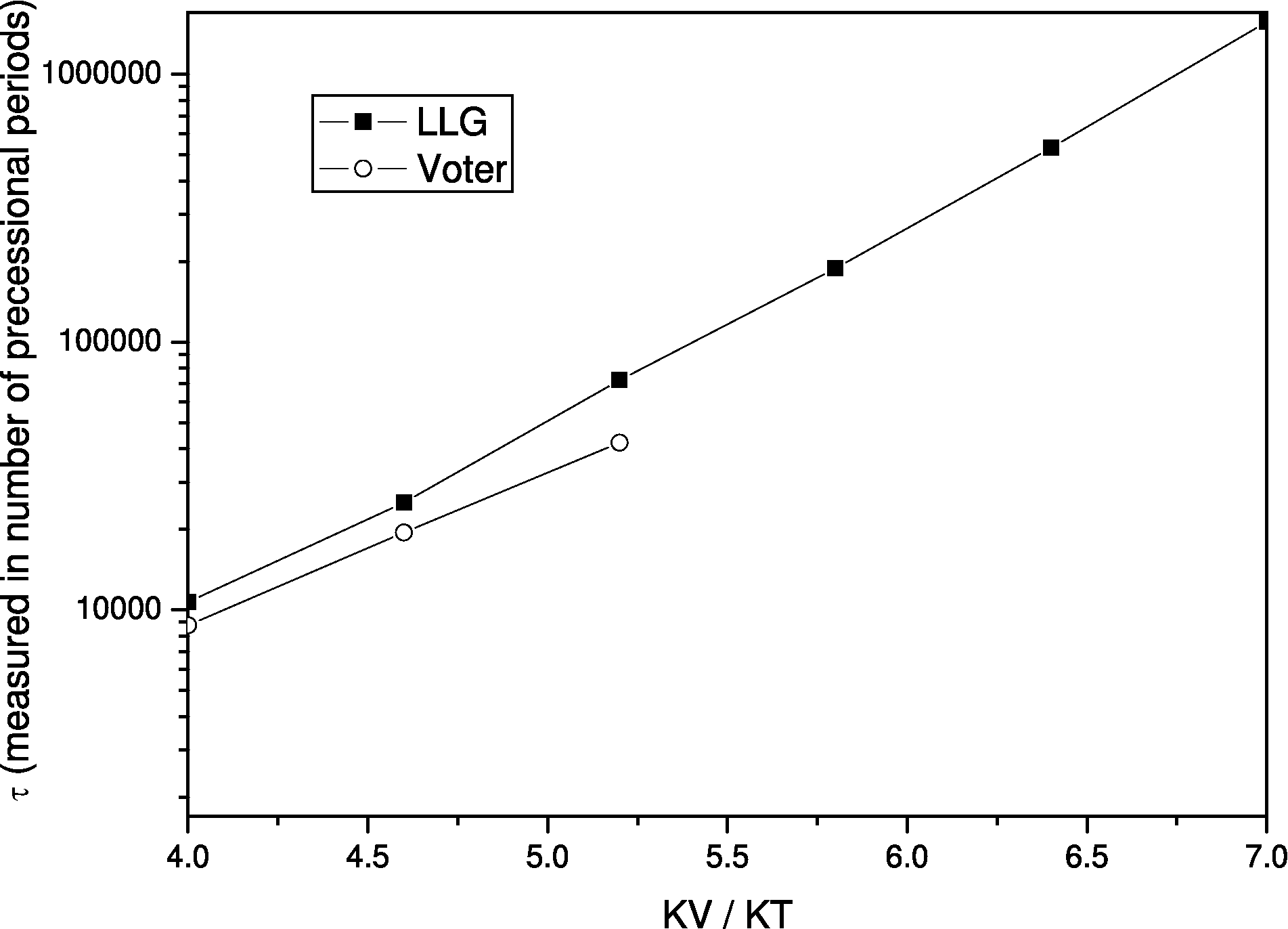In conclusion, we have implemented the method of Hyperdynamics of infrequent events'' to accelerate the molecular dynamics simulations in the case of magnetization dynamics achieving an acceleration up totimes. Higher acceleration seems also possible if one uses more sophisticated modern methods to evaluate rapidly the lowest eigenvalue of the Hessian matrix. In comparison to the time-quantified Monte Carlo, the main advantage of the method is the correct description of the influence of the precession on the thermal switching process. In contrast to the Victora method this method does not suppose a priori the Arrhenius-Néel law with one unique temperature-independent attempt frequency. It may successfully be used when various reversal modes, with different attempt frequencies, coexist during the thermal magnetization process. The limitations of the method make it useful for intermediate timescale, up to hundreds of nanoseconds, for example, for the dynamic coercivity calculations. Higher time scale seems not reachable.

2008-04-04